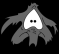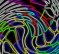## Determining the frequency (between 1-100MHz) & amplitude of a sinusoidStarted by 1 year ago12 replieslatest reply 1 year ago194 views

An acquaintance of mine asked me the following question:

We have a bandlimited signal (say 0-100 MHz) that consists of a sinusoid plus white noise. Both the amplitude and frequency of the sinusoid are unknown (but frequency is in 1-100MHz). We can also assume that in the frequency domain - there will always be a discernible peak at the injected frequency reasonably above the noise floor.  We wish to determine the frequency and amplitude of the sinusoid.

Of course, we could just sample at > 200 MSPS, do the FFT and get the information.

However, for reasons of cost and FPGA resources (we have MANY such channels in parallel) we would like to undersample and "somehow" get the above required information (i.e. frequency and amplitude of the sinusoid).

I noticed some techniques such as Compressive Sensing or Sampling and also Nyquist Folding Receiver Architecture but I do not have enough expertise to judge whether these would work in our case.

Any insights would be very much appreciated.

Clayton Loecher

[ - ]Since your bandwidth is 0 to 100MHz then you can't undersample and get frequency info.

Though you can get amplitude info wherever your frequency aliases to.

[ - ]Sounds like your client is susceptible to buzzwords.

[ - ]some questions:

• How fast is the frequency changing?
• Does it change monotonically?
• Is there truly just one frequency peak?
• what is the SNR?
• Is the noise really white (i.e. you have measured it in an actual system) or is that an assumption?
• what measurement accuracy do you need?
• how often do you need to measure the frequency?

[ - ]This is a classic task for a continuous fitting operation. I once wrote a module for this task in C that uses the minimum squares of the deviations and works iteratively. It can follow reasonable changes in both period and phase very efficiently. It also works with sine waves that contain massive harmonics. Later I transferred it to VHDL and created a core. Depending on the implementation, it is quite fast and tolerant of variations in the object parameters. The noise is the key point and determines the accuracy of the fit.

There is a core available, but for this task here, the FFT may be less resource intensive.

[ - ]If the carrier is far enough above the noise floor, you could boost the input signal into clipping and just count positive zero crossings with a counter and read/reset that counter at a given interval. The average of that value should be a little bit above the carrier frequency (some additional crossings due to noise).

Then you could subsample the (unclipped original) signal at say 10MHz, find the dominant peak (alias) and measure the amplitude. The frequency of the alias could have been caused by different original carriers, but in combination with the rough counter signal, you could determine which was the original signal frequency.

Regards

Markus

[ - ]That makes some sense if zero crossing is cared for. And is practical for FPGA platforms. It is worth trying but then you might just measure amplitude in time domain and spare any FFT.

[ - ]You can certainly undersample (aka subsample) provided that the signal bandwidth is narrower than the sample rate.  It's done all the time, creating Nyquist zones.  You need to filter out the images after subsampling.  Frequency translation of the filtered signal to baseband is recommended.  Once at baseband there are many ways to extract frequency and amplitude, depending on the desired accuracy and measurement rate.  Interpolation followed by an FFT works well.

[ - ]Every disclaimer for ignorance is claimed ...

Given that the desired signal is a fixed frequency sinusoid whose power exceeds (by some amount) the noise, could you not undersample and determine frequency by comparing detected aliased frequencies?  I'm not sure if this saves much hardware.  Maybe it could be part of a per-channel pipeline of detection.

Coop

aa1ww

[ - ]You can try the Sparse Fourier Transform.

For a single sinusoid as in your case, you might get by with just the Chinese Remainder Theorem. Your approach would go something like:

- Assume some sample rate >200 MSPS

- The combination of the above two steps gives the number of ideal FFT bins (i.e. number of samples), N (could be large)

- Find K coprime integers N_1,N_2,...N_K such that their product is approximately N (let's say it's exact N=N_1 x N_2 x ...)

- Do K FFTs of lengths N_1,N_2,... (sample strides are N/N_1,N/N_2,... respectively)

- Find the peak bin k_i in each FFT of length N_i. Assuming k is the bin of your sinusoid for the ideal full FFT of length N, you then have a system of equations

k = k_1 (mod N_1)

k = k_2 (mod N_2)

etc.

See https://en.wikipedia.org/wiki/Chinese_remainder_th... to recover k.

[ - ]Remember this is fpga platform and main theme is centred on simple resource efficient solution. If I can sample at correct rate and do one fft there is no issue but OP is asking for simpler solutions.

[ - ]